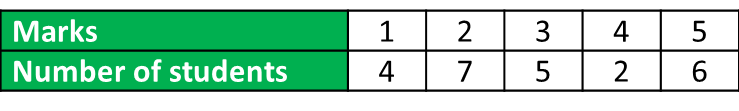# 7.2.4 Interquartile Range of Grouped Data

Interquartile Range of Grouped Data (without Class Interval)

Example 2:
The table below shows the marks obtained by a group of Form 4 students in school mid-term science test.Determine the interquartile range of the distribution.

Solution:
Lower quartile, Q1 = the   $\frac{1}{4}{\left(24\right)}^{\text{th}}$ observation
= the 6thobservation
= 2

Upper quartile, Q3 = the   $\frac{3}{4}{\left(24\right)}^{\text{th}}$ observation
= the 18thobservation
= 4Interquartile Range
= upper quartile – lower quartile
= Q3Q1
= 4 – 2
= 2